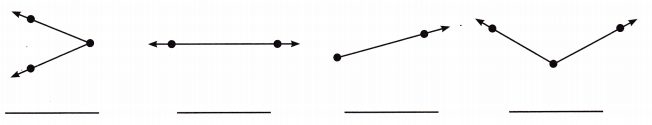# McGraw Hill Math Grade 4 Chapter 12 Lesson 2 Answer Key Angles

Practice the questions of McGraw Hill Math Grade 4 Answer Key PDF Chapter 12 Lesson 2 Angles to secure good marks & knowledge in the exams.

## McGraw-Hill Math Grade 4 Answer Key Chapter 12 Lesson 2 Angles

Solve.

Question 1.
What is an angle?
An angle is a figure formed by two rays that share the same endpoint.

Question 2.
What is a vertex?
Vertex is endpoint of an angle.

Question 3.
Identify the figures below.Explanation:
I identified the figures and labeled them
They are an angle, a line, a ray and an angle respectively.

Question 4.
Draw ∠EFG. Be sure to write the letters E, F, and G at the correct points.